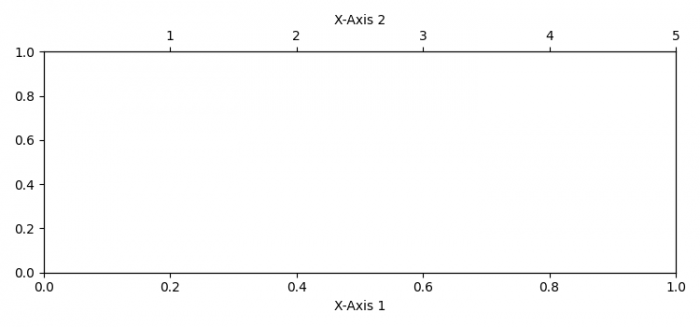# How to add a second X-axis at the bottom of the first one in Matplotlib?

To add a second X-axis at the bottom of the first one in Matplotlib, we can take the following

## Steps

• Set the figure size and adjust the padding between and around the subplots.
• Get the current axis (ax1) using gca() method.
• Create a twin axis (ax2) sharing the Y-axis.
• Set X-axis ticks at Axis
• Set X-axis labels at Axis 1 and
• To display the figure, use show() method.

## Example

import matplotlib.pyplot as plt

plt.rcParams["figure.figsize"] = [7.50, 3.50]
plt.rcParams["figure.autolayout"] = True

ax1 = plt.gca()
ax2 = ax1.twiny()

ax2.set_xticks([1, 2, 3, 4, 5])
ax1.set_xlabel("X-axis 1")
ax2.set_xlabel("X-axis 2")

plt.show()

## Output